PSAT Math : How to find an angle in a polygon

Example Questions

2 Next →

Example Question #106 : Plane Geometry

Pentagon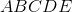is regular. If diagonal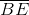is drawn, which of the following describes Quadrilateral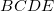?

Quadrilateralis a parallelogram but neither a rectangle nor a rhombus.

Quadrilateralis a rhombus but not a rectangle.

None of the other responses is correct.

Quadrilateralis a trapezoid.

Quadrilateralis a rectangle but not a rhombus.

Quadrilateralis a trapezoid.

Explanation:

The figure described is below.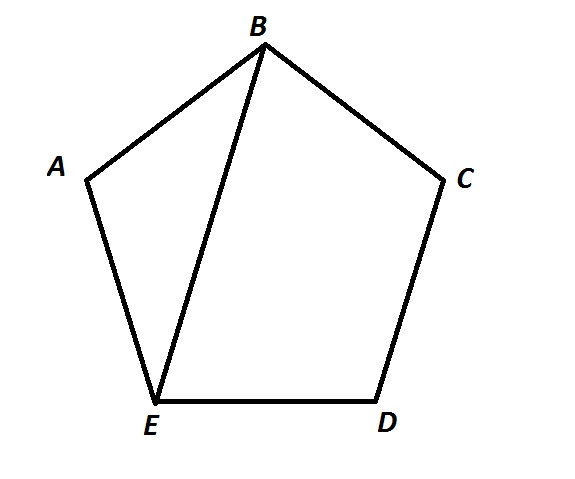Each of the angles of the pentagon has measure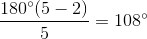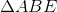is an isosceles triangle, and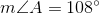, so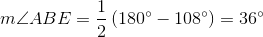and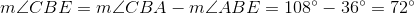Since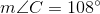,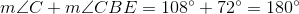and by the parallel postulate,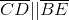Quadrilateralhas exactly one pair of parallel sides, so it is a trapezoid.

2 Next →